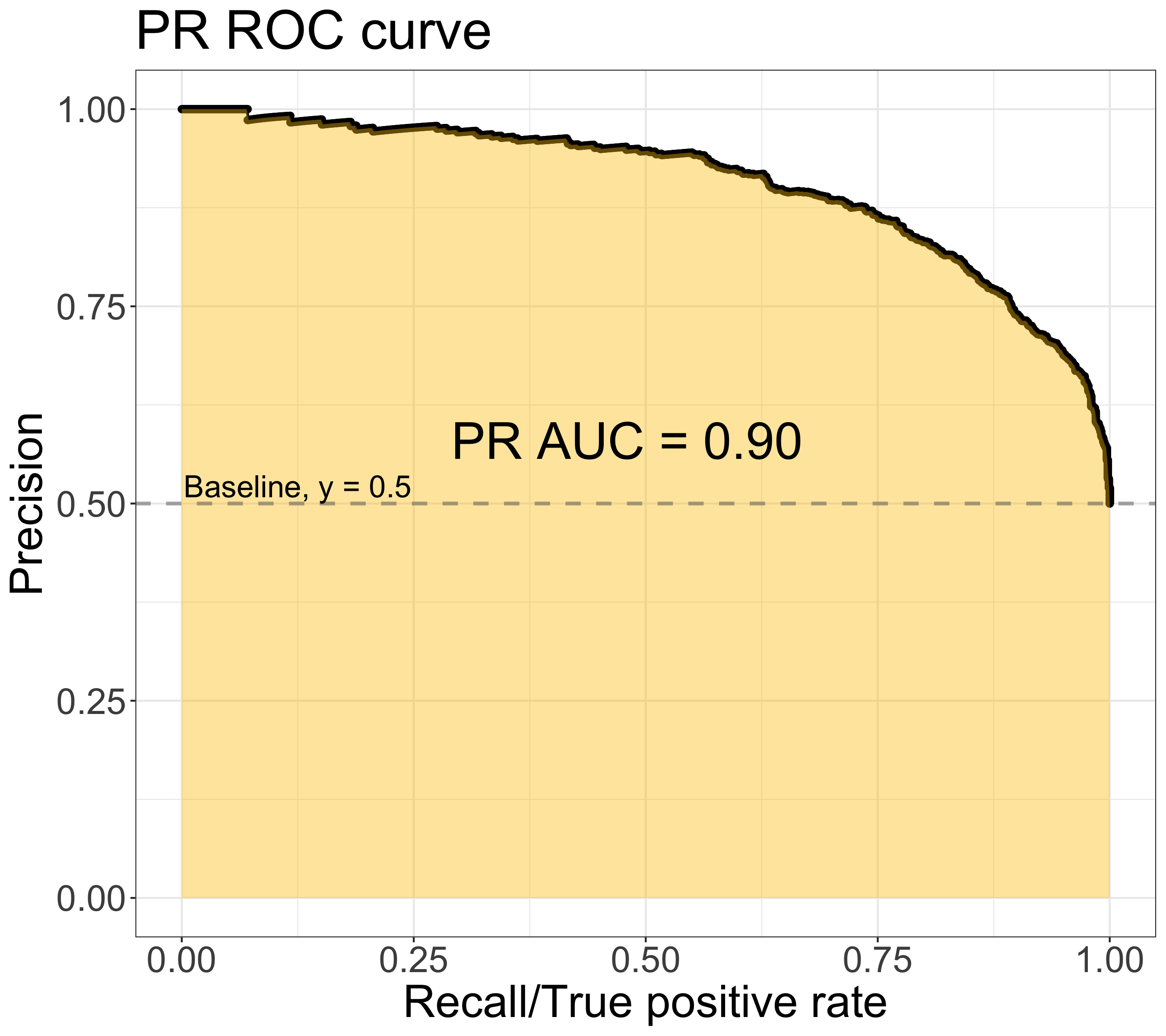# Compute the AUC of Precision-Recall Curve

2020-04-25
Compute the AUC of Precision-Recall Curve

After the theory behind precision-recall curve is understood (previous post), the way to compute the area under the curve (AUC) of precision-recall curve for the models being developed becomes important. Thanks to the well-developed scikit-learn package, lots of choices to calculate the AUC of the precision-recall curves (PR AUC) are provided, which can be easily integrated to the existing pipeline of models.

# Which function computes the PR AUC?

At first glance of the list in the metrics module in scikit learn, the only function that seems related to precision-recall curve is metrics.precision_recall_curve. However, it computes the values of the curve rather than the area under the curve (AUC). First, the plot will have to be constructed, and next step is to compute the PR AUC using metrics.auc. Is there any other way to get the PR AUC in simply one step? After intense search on the internet, I found that metrics.average_precision_score actually is the function I am looking for. But why? Is average precision equal to PR AUC? Let’s explore it in the following section.

# What’s average precision?

Average precision computes the average value of precision over the interval from recall = 0 to recall = 1. precision = p(r), a function of r - recall:

$Average\ Precision = \int_{0}^{1} p(r)dr$

Does this formula give clues about what average precision stands for? Let’s look into a precision-recall curve.The integral computes the area under the precision-recall curve - the yellow area. It means that the average precision is equal